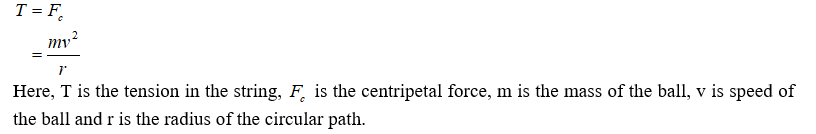# A 3.5 kg ball is traveling in a circle of radius 1 m at a constant speed of 9 m/s. What is the tension (in N) in the string?

Question
1 views

A 3.5 kg ball is traveling in a circle of radius 1 m at a constant speed of 9 m/s. What is the tension (in N) in the string?

check_circle

Step 1

The tension in the string must be equal to the centripetal force on the ball. It is given as,...

### Want to see the full answer?

See Solution

#### Want to see this answer and more?

Solutions are written by subject experts who are available 24/7. Questions are typically answered within 1 hour.*

See Solution
*Response times may vary by subject and question.
Tagged in